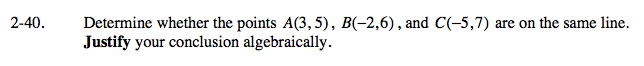### Home > AC > Chapter 14 > Lesson 14.2.1.3 > Problem2-40

2-40.

Determine whether the points A(3, 5) , B(−2, 6) , and C(−5, 7) are on the same line. Justify your conclusion algebraically. Homework Help ✎Find the slope between points A and B.

$\frac{6-5}{-2-3}=-\frac{1}{5}$

Now find the slope between points B and C.

$\frac{7-6}{-5+2}=-\frac{1}{3}$